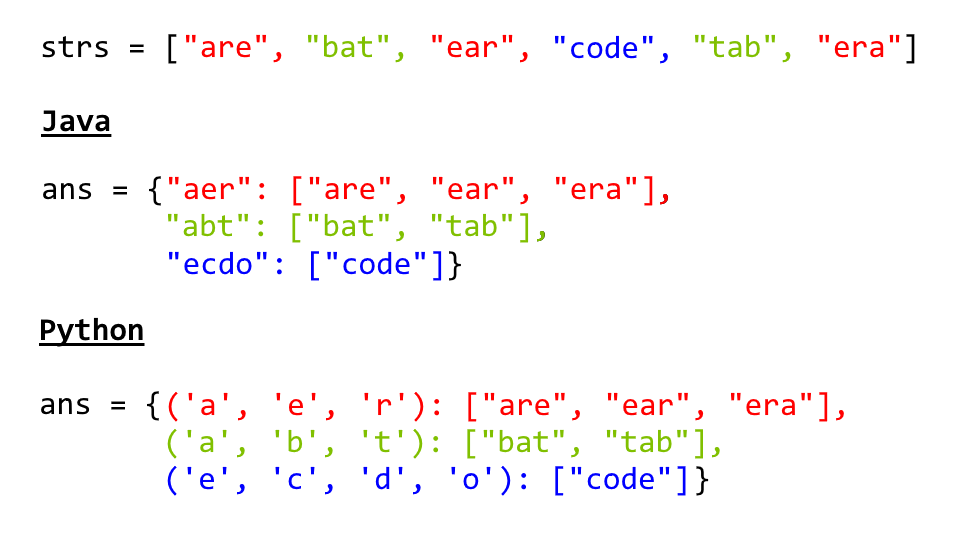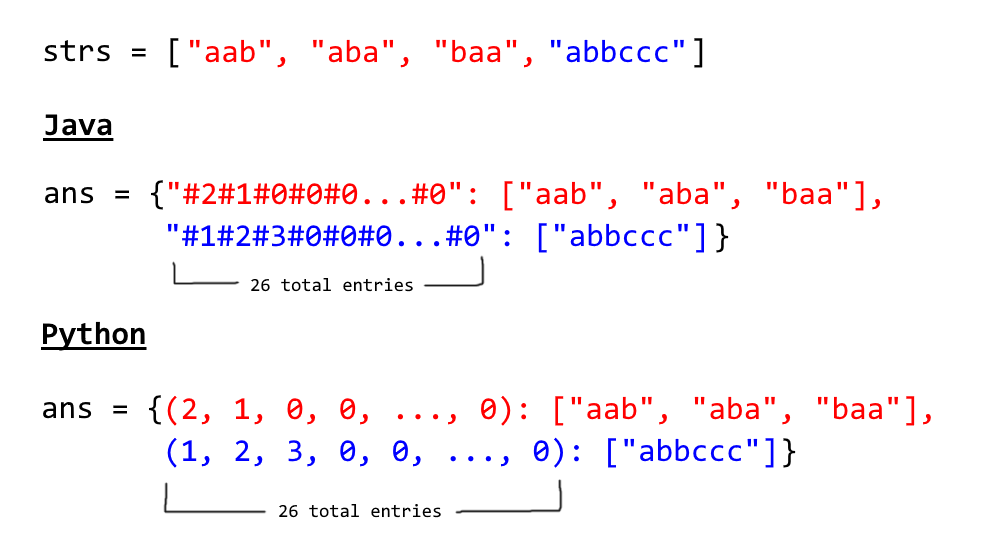# leetcode题解之49. 字母异位词分组

输入: ["eat", "tea", "tan", "ate", "nat", "bat"]

[
["ate","eat","tea"],
["nat","tan"],
["bat"]
]

• 所有输入均为小写字母。
• 不考虑答案输出的顺序。

#### 方法一：排序数组分类class Solution {
public List<List<String>> groupAnagrams(String[] strs) {
if (strs.length == 0) return new ArrayList();
Map<String, List> ans = new HashMap<String, List>();
for (String s : strs) {
char[] ca = s.toCharArray();
Arrays.sort(ca);
String key = String.valueOf(ca);
if (!ans.containsKey(key)) ans.put(key, new ArrayList());
}
return new ArrayList(ans.values());
}
}

class Solution(object):
def groupAnagrams(self, strs):
ans = collections.defaultdict(list)
for s in strs:
ans[tuple(sorted(s))].append(s)
return ans.values()


https://www.jianshu.com/p/bb4a067ae437

• 时间复杂度：$O(NK \log K)$，其中 $N$strs 的长度，而 $K$strs 中字符串的最大长度。当我们遍历每个字符串时，外部循环具有的复杂度为 $O(N)$。然后，我们在 $O(K \log K)$ 的时间内对每个字符串排序。

• 空间复杂度：$O(NK)$，排序存储在 ans 中的全部信息内容。

#### 方法二：按计数分类class Solution {
public List<List<String>> groupAnagrams(String[] strs) {
if (strs.length == 0) return new ArrayList();
Map<String, List> ans = new HashMap<String, List>();
int[] count = new int;
for (String s : strs) {
Arrays.fill(count, 0);
for (char c : s.toCharArray()) count[c - ‘a‘]++;
StringBuilder sb = <span class="hljs-keyword">new</span> StringBuilder(<span class="hljs-string">""</span>);
<span class="hljs-keyword">for</span> (<span class="hljs-keyword">int</span> i = <span class="hljs-number">0</span>; i &lt; <span class="hljs-number">26</span>; i++) {
sb.append(<span class="hljs-string">‘#‘</span>);
sb.append(count[i]);
}
String key = sb.toString();
<span class="hljs-keyword">if</span> (!ans.containsKey(key)) ans.put(key, <span class="hljs-keyword">new</span> ArrayList());
}
<span class="hljs-keyword">return</span> <span class="hljs-keyword">new</span> ArrayList(ans.values());
}

}


class Solution:
def groupAnagrams(strs):
ans = collections.defaultdict(list)
for s in strs:
count =  * 26
for c in s:
count[ord(c) - ord(‘a‘)] += 1
ans[tuple(count)].append(s)
return ans.values()


• 时间复杂度：$O(NK)$，其中 $N$strs 的长度，而 $K$strs 中字符串的最大长度。计算每个字符串的字符串大小是线性的，我们统计每个字符串。

• 空间复杂度：$O(NK)$，排序存储在 ans 中的全部信息内容。

leetcode题解之49. 字母异位词分组

(0)
(0)